# 幻世录1攻略隐藏物品位置 幻世录1攻略隐藏物品汇总

幻世录1攻略隐藏物品在哪？游戏中有许多隐藏物品需要玩家去寻找，下面小编为大家带来幻世录1攻略隐藏物品汇总，感兴趣的小伙伴一起了解一下吧。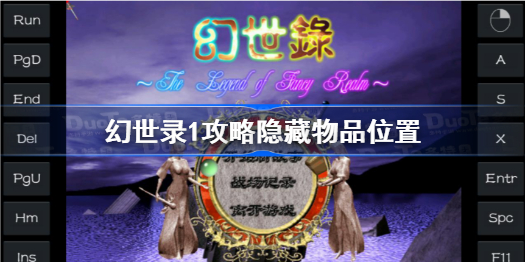### 幻世录1攻略隐藏物品在哪

在桌面游戏启动的快捷方式中，属性的目标项中加入-pos(需注意-前面加空格)的密技才可看到坐标。

双头的死神(欧姆村)

X=656，Y=560

玻璃戒指、回复药、解毒草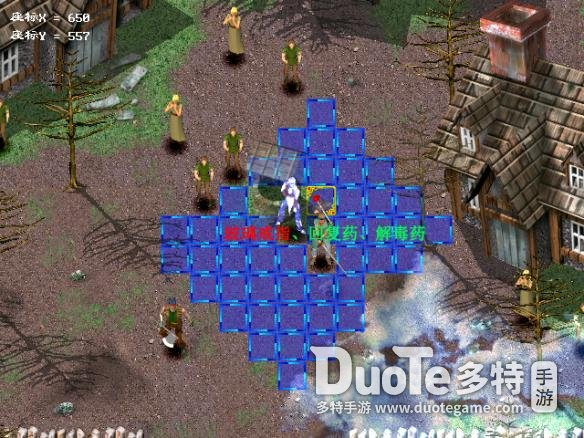遭遇(戈尔山道)

X=496，Y=464

阔刃剑、回复药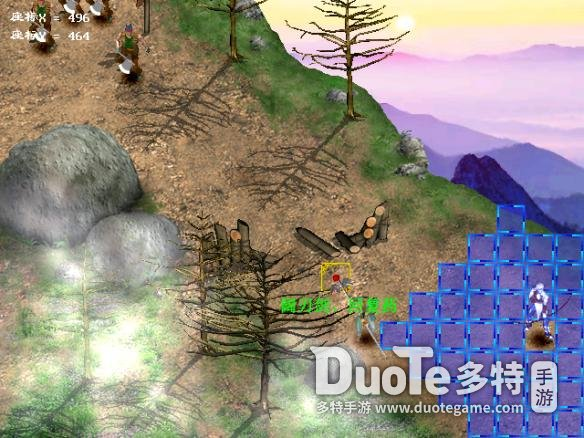X=176，Y=464

妖精之泉x2、御之源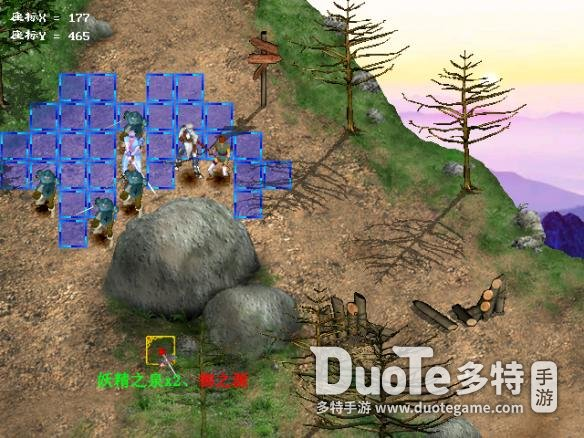巢窟(盗贼洞窟)

X=624，Y=368

黄金首饰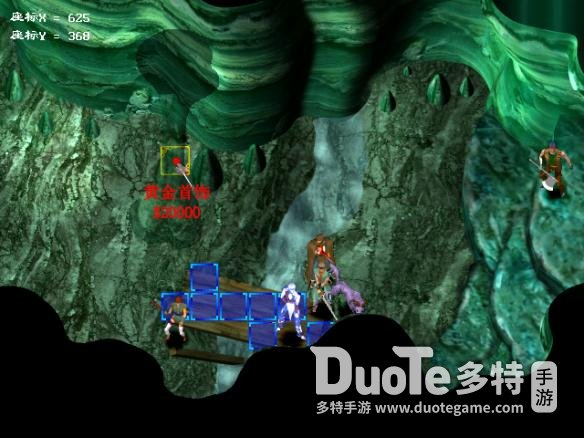猛拧(呼啸平原)

X=304，Y=592

土之源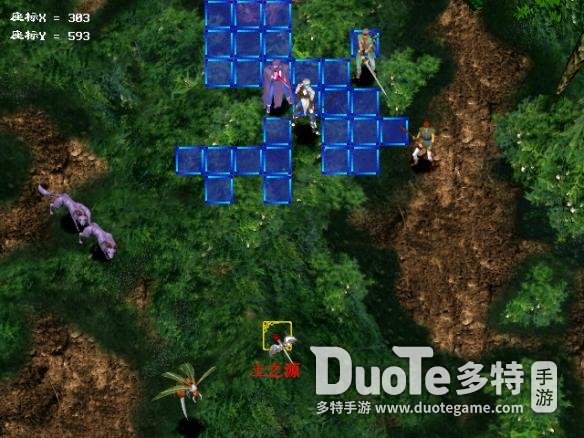宣战(席达镇)

X=400，Y=336

魔之源、黄金首饰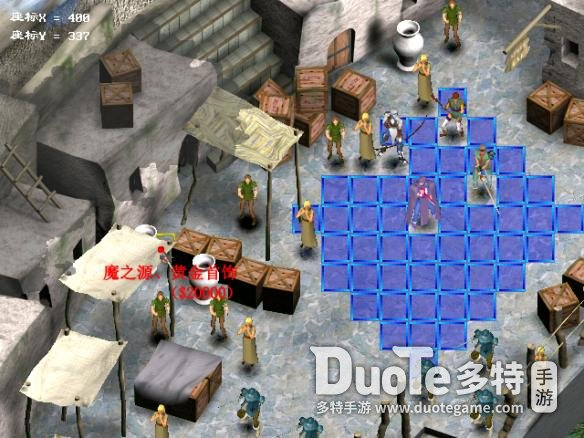X=816，Y=688

烈炎戒指、力之源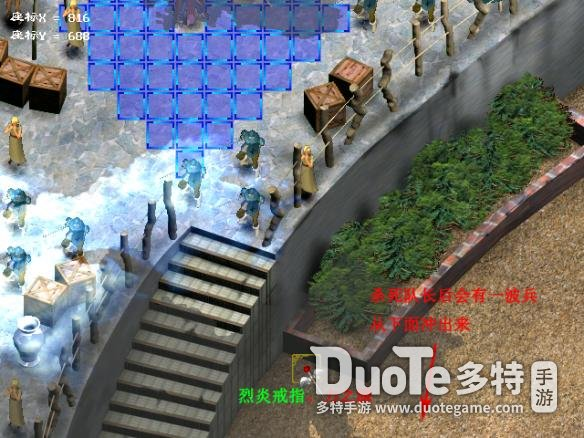寂寥(宁静之森)

X=592，Y=592

银制发饰、解毒草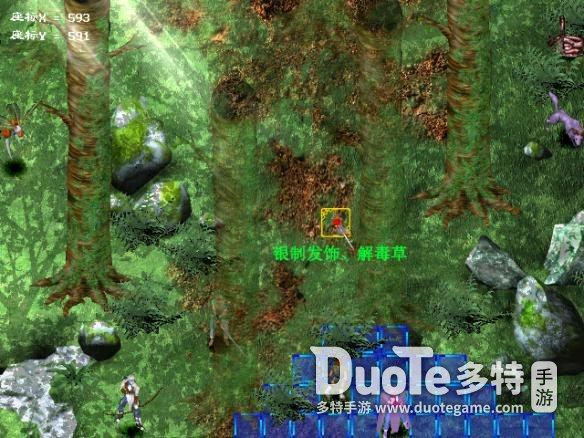X=784，Y=368

凝气之环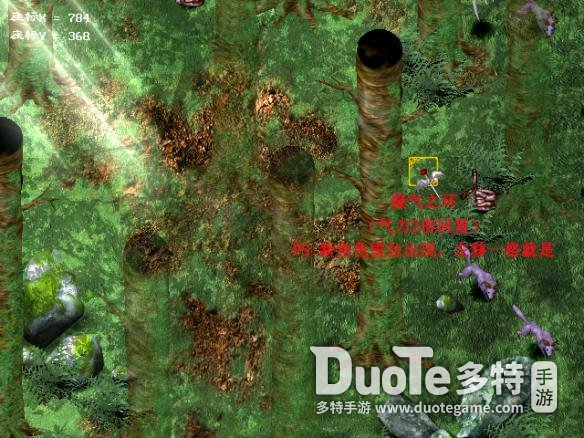哀怨(帕尼西雅城废墟)

X=816，Y=240

水晶剑、琥珀水晶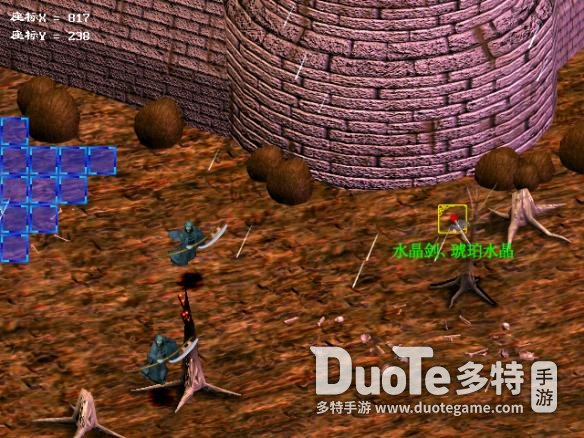狙兵(菲纳斯河畔)

X=496，Y=304

叁叉戟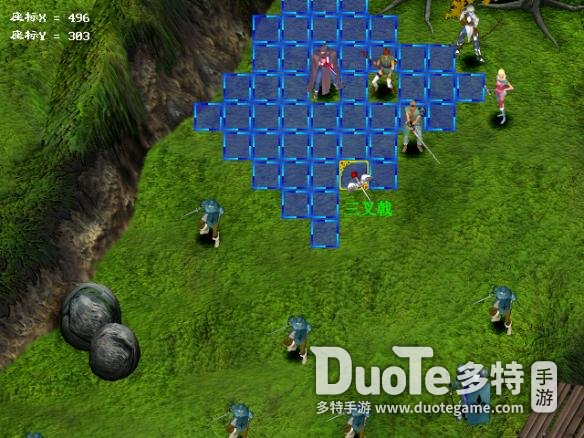X=1392，Y=144

风之源、水之源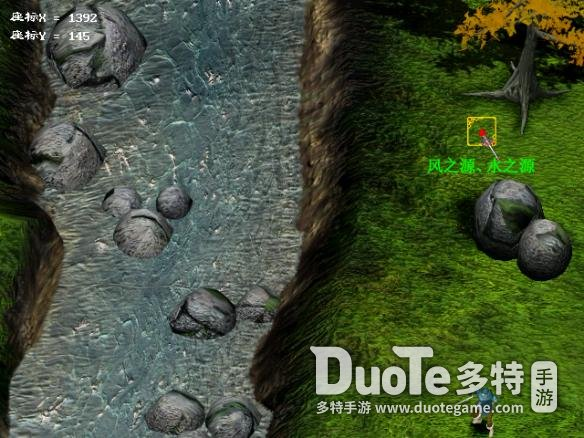失落(废都曼多力亚)

X=1296，Y=560

黄金的圣杯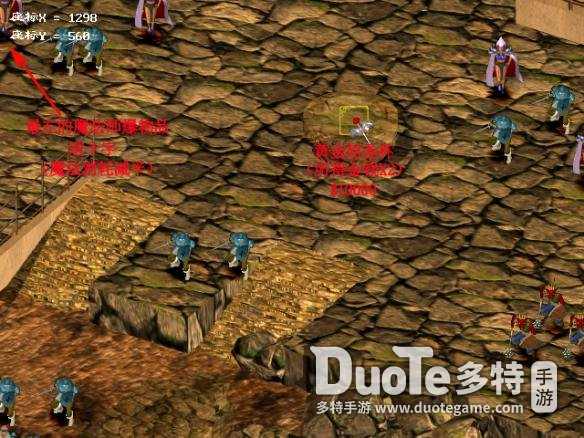这一关之后，将有个选择，决定后面两个关获得什么样的隐藏物品。

到了薛维斯港，到港口找搬运工人交谈，得知商人克里夫有通行证，再与船长交谈，得知命运神殿的事，这时有二个选择：一、去艾瓦台地追克里夫，二、去命运神殿。选取前者接本关“援救”，选后者从命运神殿回到艾瓦台地时克里夫会被杀，接“寻”。

去过神殿和没去神殿区别就在于：是获得奥义之证(攻击距离+1)，还是获得逆十字(魔法消耗减半)。

援救(艾瓦台地)

X=624，Y=144

逆十字

X=432，Y=560

火红水晶、灵之源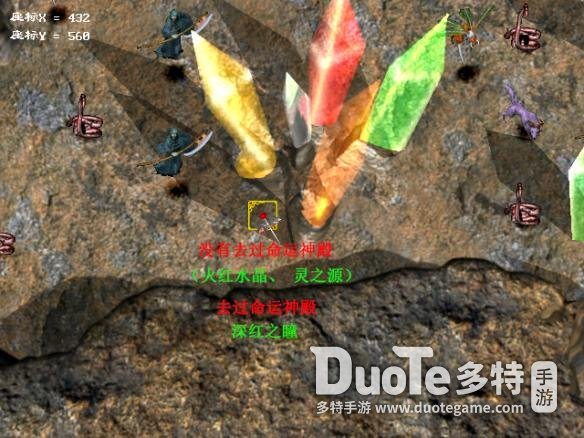寻(艾瓦台地)

X=624，Y=144

湛蓝水晶、土之源

X=432，Y=560

深红之瞳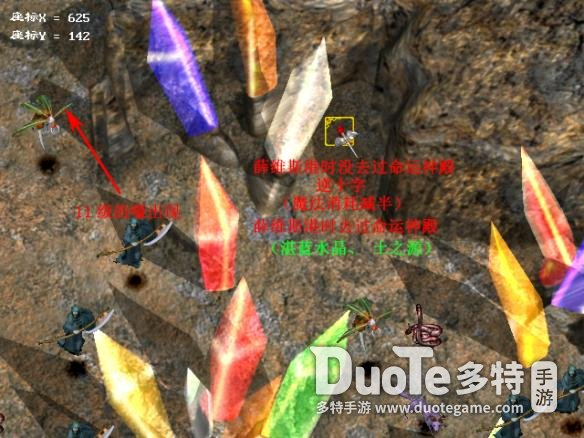PS:如果是“寻”，则通行证在货箱周围，有的说在货箱周围四角，有的说在上面两角。全凭运气，反正就在周围紧挨货箱的格子里。

真理(利鲁玛山地)

※克里夫未死：

X=48，Y=80

幸运缎带

X=1488，Y=208

凝气之环

X=592，Y=720

秘药、妖精之泉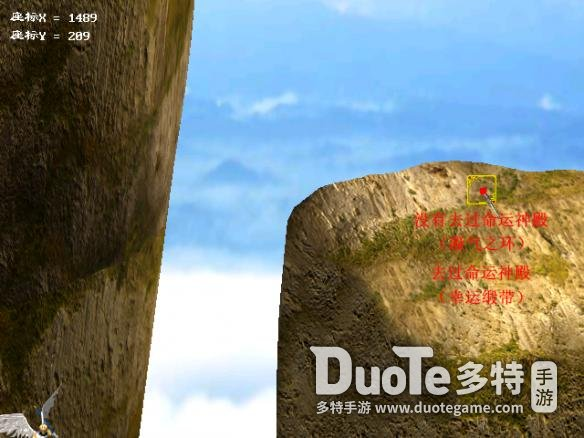※克里夫死亡：

X=48，Y=80

凝气之环

X=1488，Y=208

幸运缎带

X=432，Y=944

奥义之证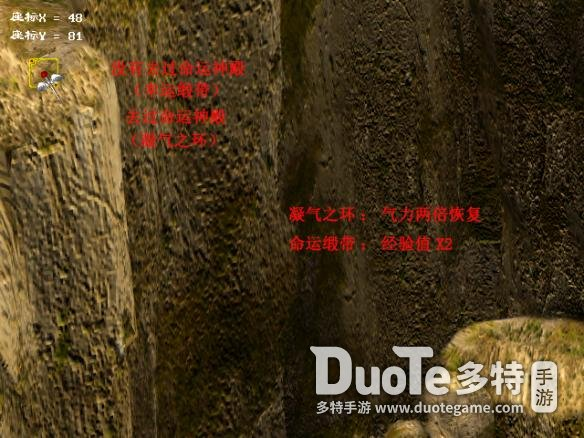※克里夫未死：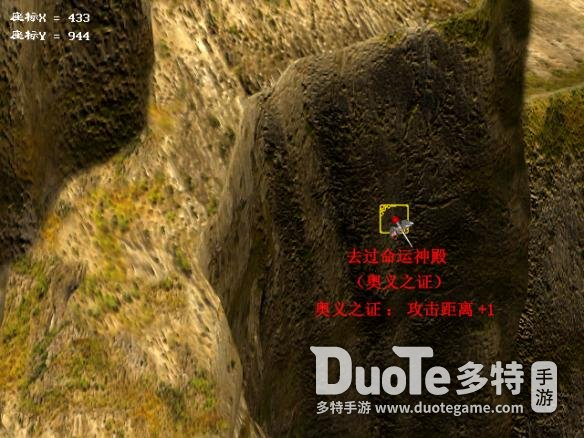※克里夫死亡：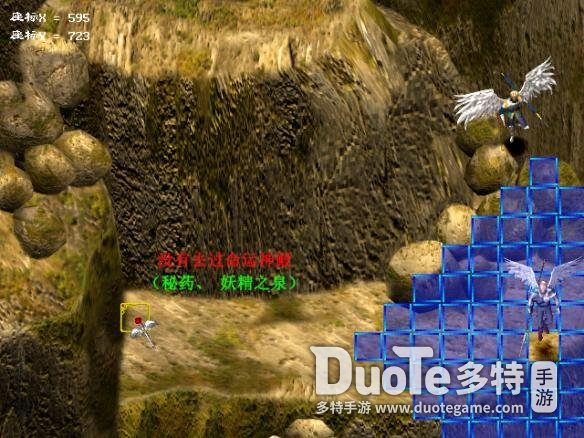魔骑士(回音之谷)

X=144，Y=48

刚岩之盔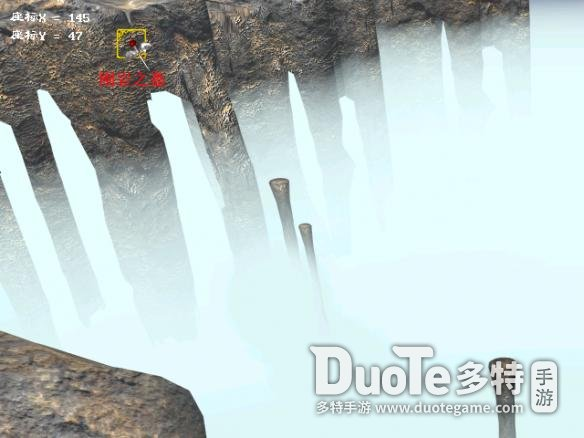X=144，Y=432

琥珀水晶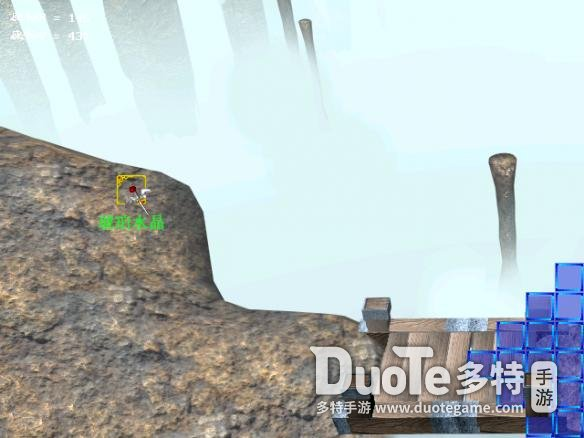这一关脱离和打跑克罗蒂，只是决定后面一关卡隐藏物品位置的不同而已。

狂砂(哈莫特沙漠)

※回音之谷打倒克罗蒂：

X=592，Y=912

女神之冠

※回音之谷脱离：

X=112，Y=656

女神之冠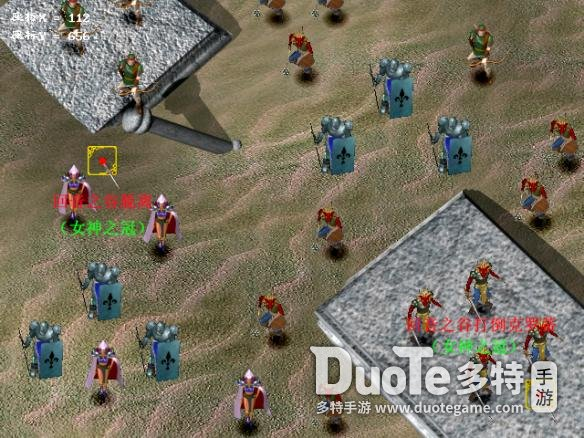信念(约瑟河)

X=912，Y=304

翠绿水晶、水之源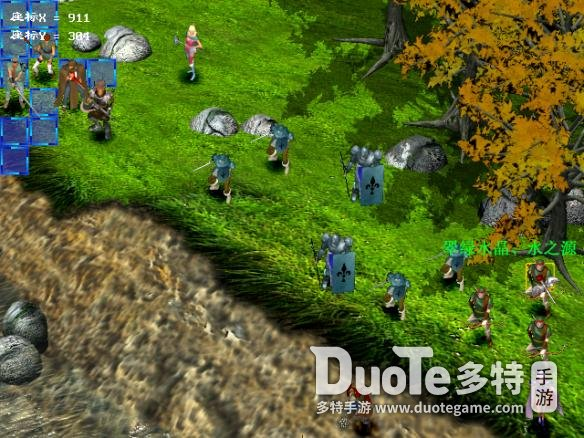X=144，Y=624

极光护轮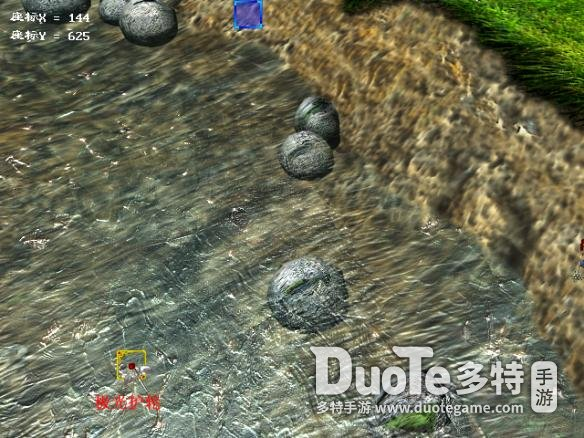打完这一关，基本全员属性都黄了，可以回去转职了。再往前走的关卡，将无法回转，所以如果还有队员属性没黄，只能回头练级。

分支：怒涛(巴瀚纳海峡)

X=1360，Y=1552

冥晦之轮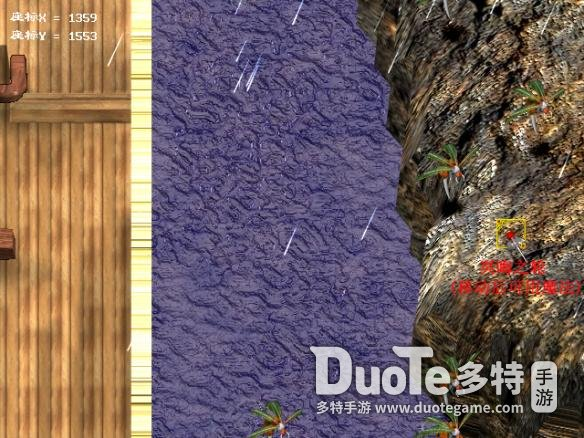分支：异种(深渊之沼)

X=752，Y=48

绿结晶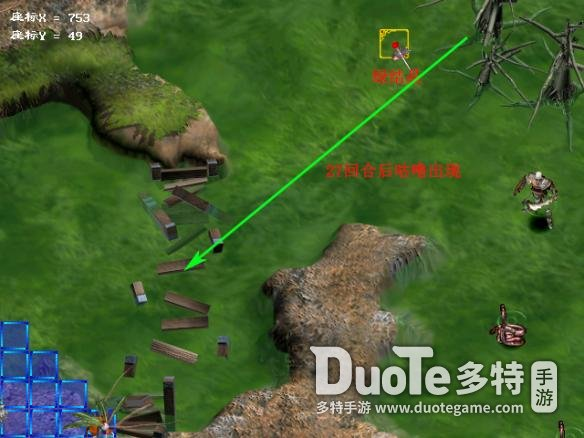离散(绝望之谷)

X=752，Y=176

火之源、风之源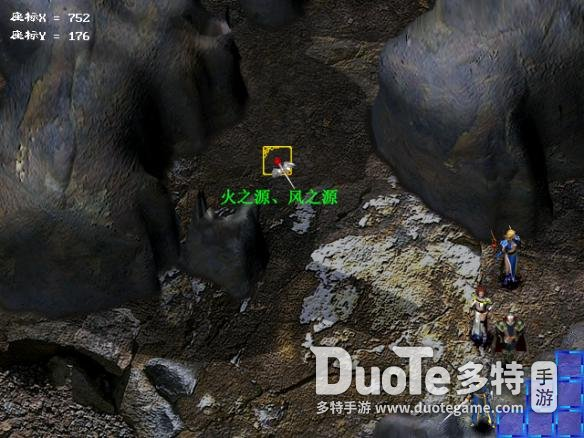X=336，Y=48

大地之衣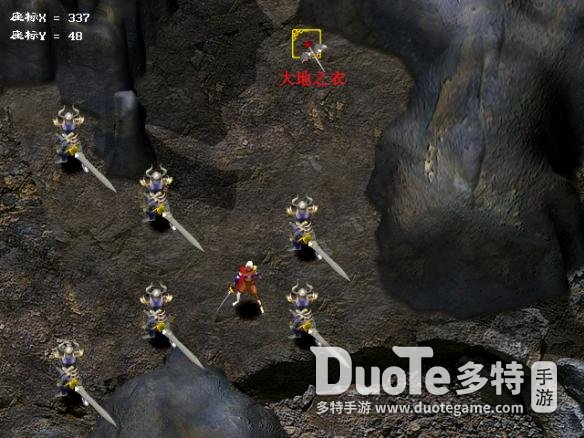奔逃(漆黑之森)

X=176，Y=560

邪眼假面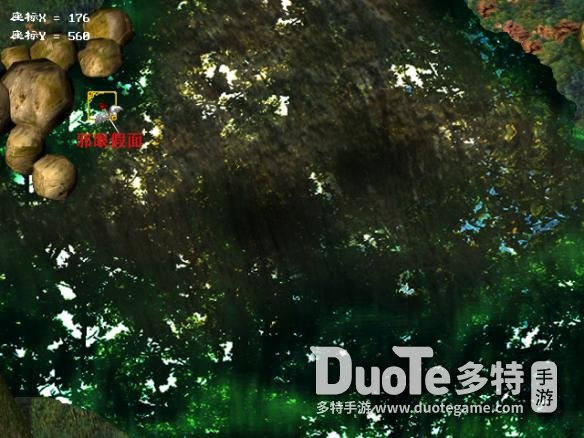X=592，Y=496

湛蓝水晶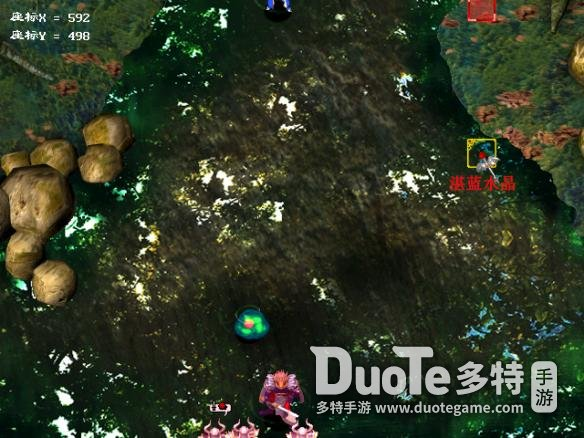解明(黄昏之丘阴)

X=240，Y=304

黑水晶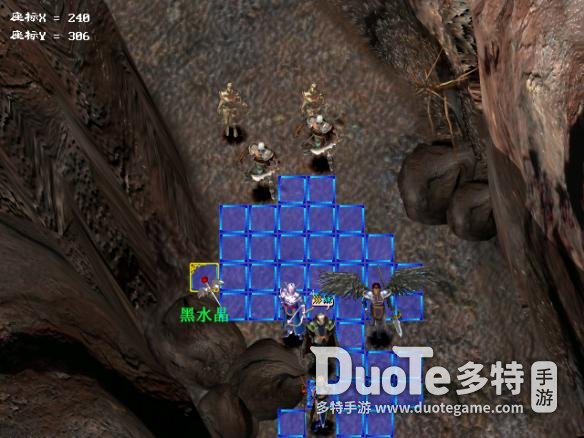阻碍(拉格纳沼地)

X=336，Y=688

翠绿水晶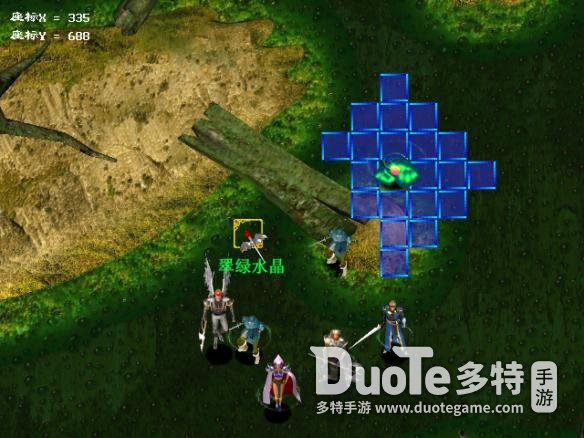虚实(沙罗尼亚近郊)

X=576，Y=704

丈八蛇矛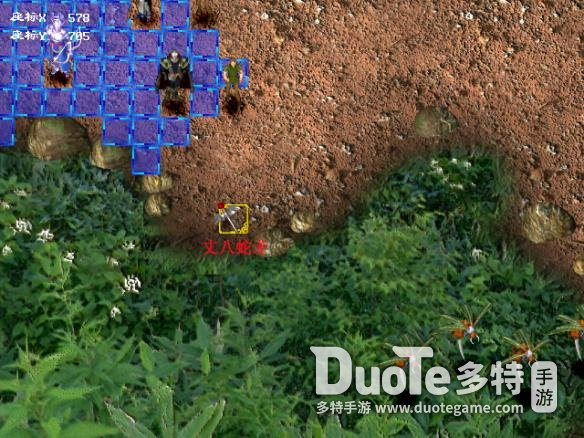X=640，Y=160

琥珀水晶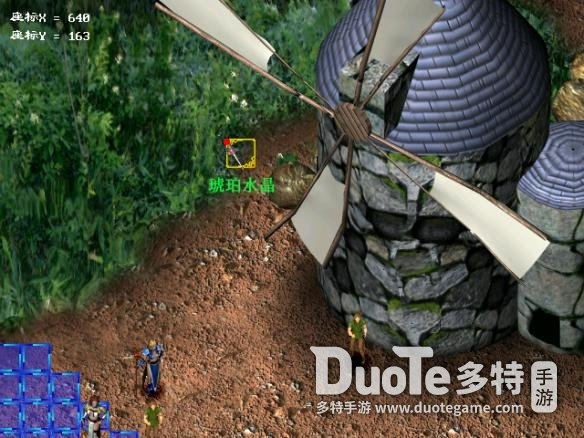变节(萨鲁司海岸)

X=304，Y=560

火红水晶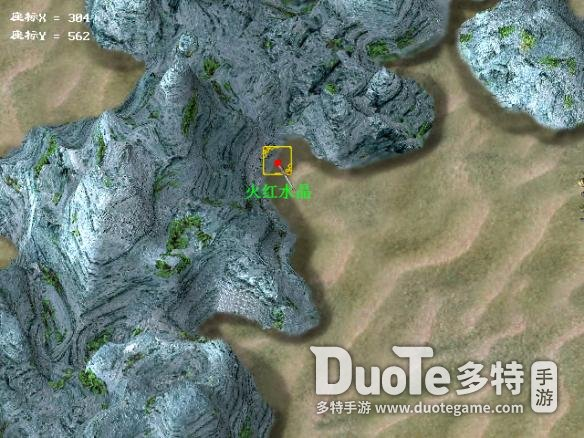X=112，Y=1072

奥义之证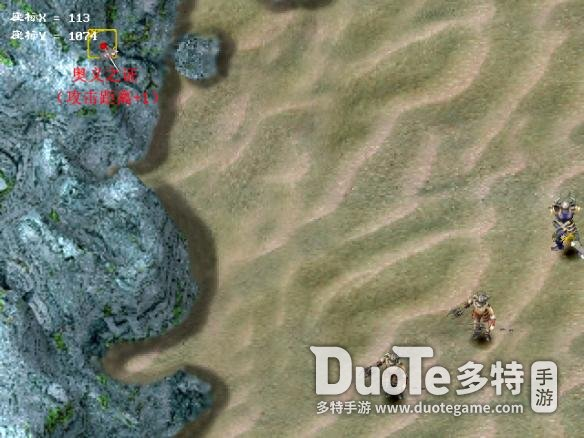未知(古代神殿遗迹)

X=272，Y=144

黄金的圣杯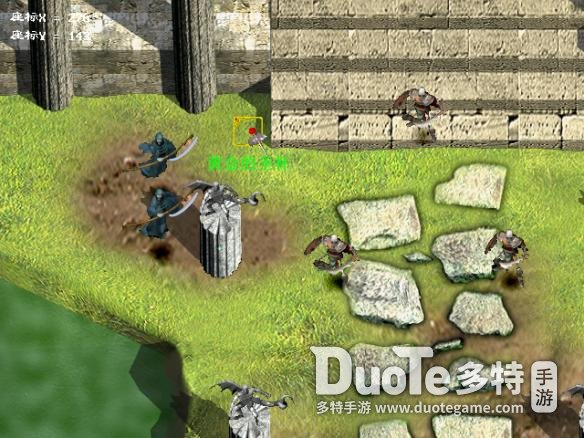崩陷(黄昏之丘阳)

X=112，Y=272

隐密之轮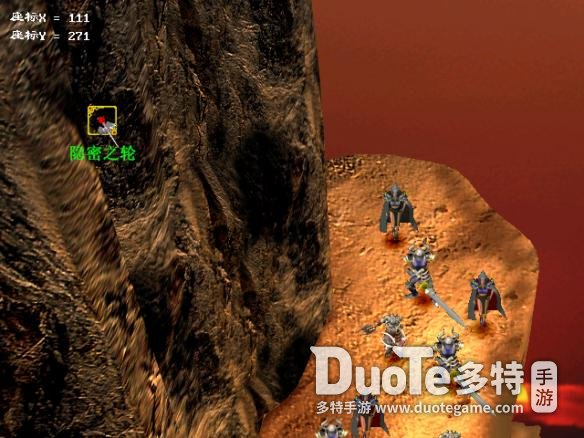恶魔之翼(悲叹之湖)

X=80，Y=560

圣骑士勋章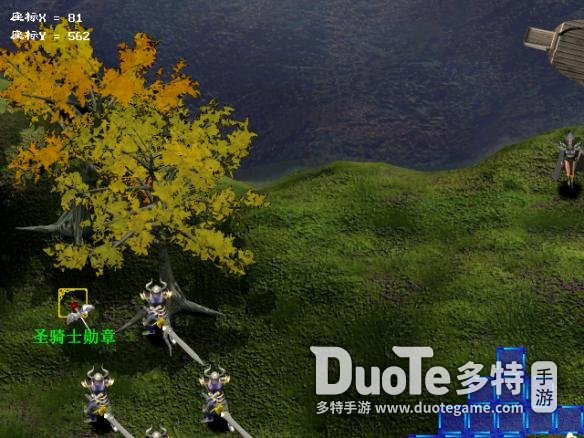X=1200，Y=880

支配者之杖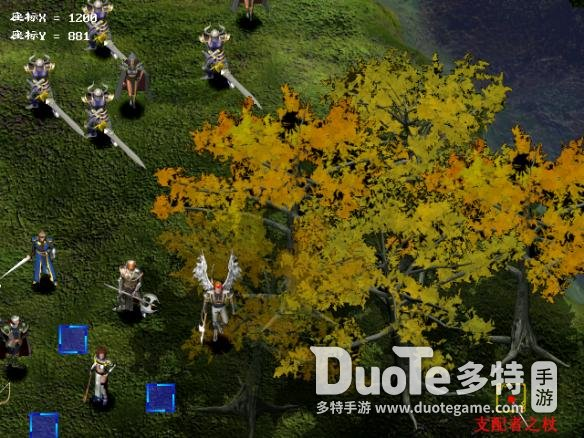开始出现分支，我个人觉得先去瀑布比较好，因为有个加移动距离的装备，可以用在精灵宝库里;而且去精灵族和去瀑布打龙中间，运气好，可以不遇怪。

猎杀(尼布鲁瀑布)

X=912，Y=240

恶魔晶石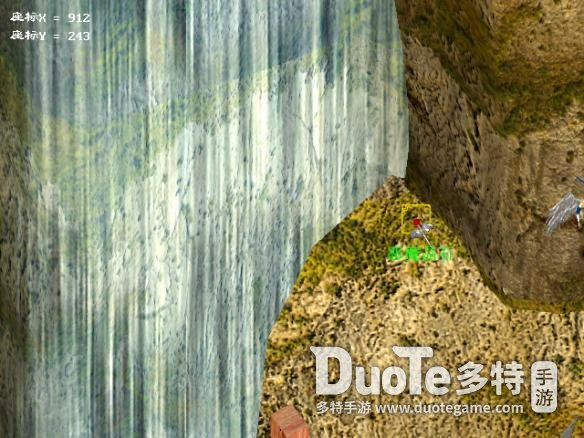X=1168，Y=976

追风之羽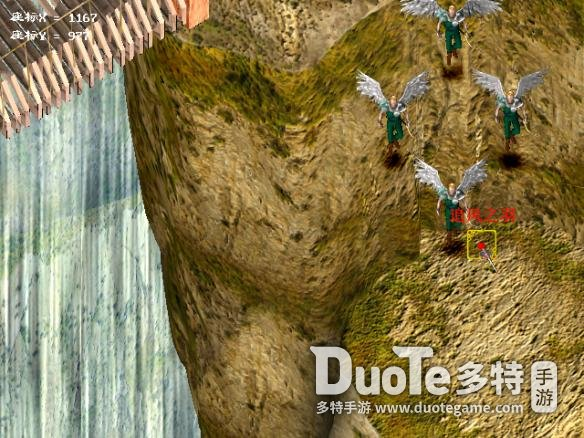破浪(日没湾)

X=80，Y=496

海龙之弓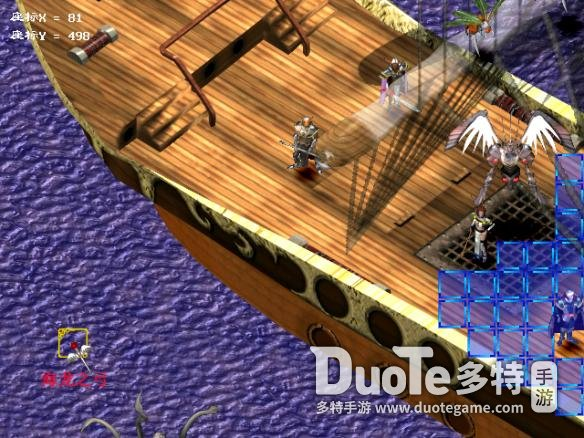遗产(众神的宫殿遗址)

X=880，Y=752

白光之翼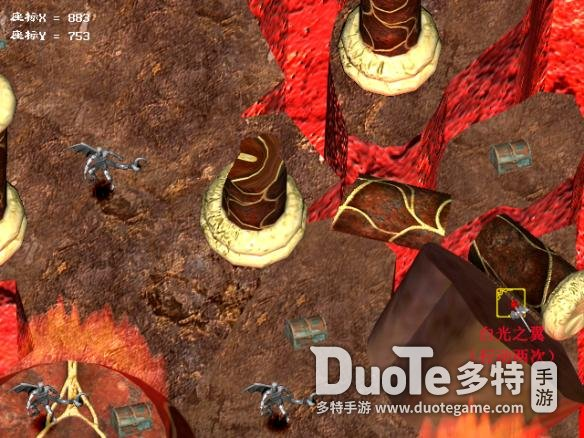X=528，Y=1872

穹苍之链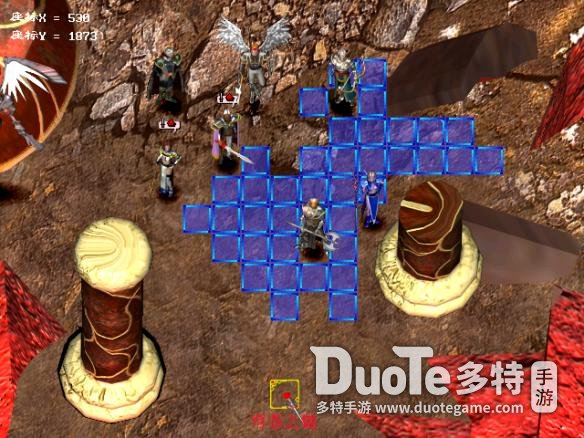分支：深邃之间(幽暗坟场)

X=272，Y=656

星尘之冠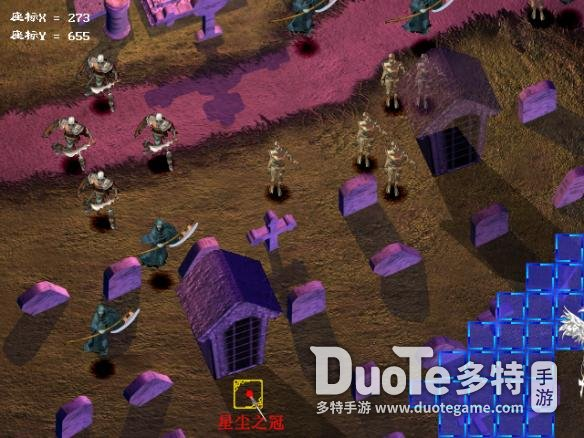分支：辉煌(龙之息)

X=48，Y=816

舞空之靴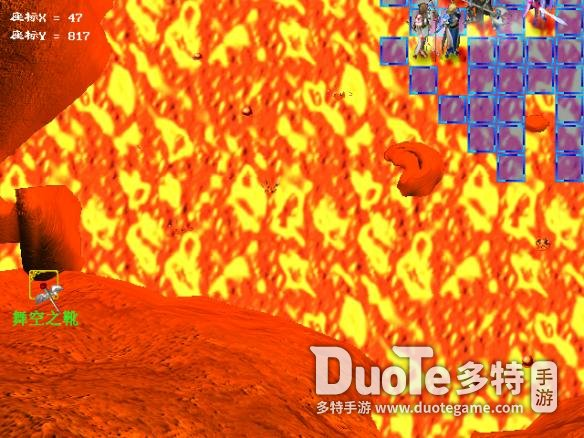残光(亚修顿大桥)

X=1104，Y=1008

旭日之盔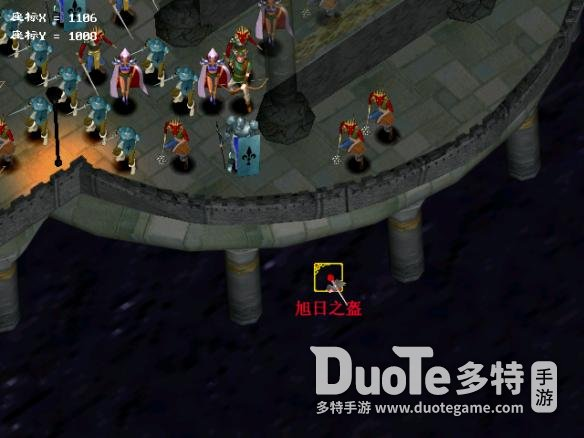游戏一共有三种结局：

一、是打倒魔神地劫神;

二、是打倒毁灭天使;

三、是打倒咕噜最终型态。

简单的说，可分为：

一、放过席德尔：A.咕噜未转职，结局为二。B.咕噜转职，结局为二或三(看分数高低)。

二、杀死席德尔:A咕噜未转职，结局为一。B.咕噜转职，结局为三。

幻世录1攻略隐藏物品汇总已经分享在上面，感兴趣的小伙伴可以参考本篇攻略找到隐藏物品，希望能为各位玩家们带来帮助，想要了解更多游戏攻略及资讯请持续关注多特手游。